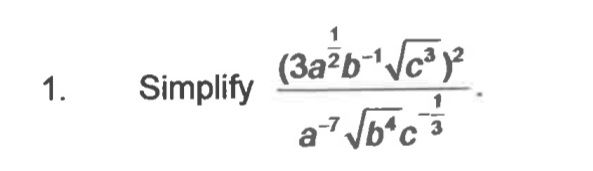User Name Remember Me? Password

 Algebra Pre-Algebra and Basic Algebra Math Forum

 January 8th, 2017, 05:49 AM #1 Member   Joined: Nov 2016 From: Ireland Posts: 84 Thanks: 3 Simplify the monster Ok so I woke up this morning to find this thing at the bottom of my bed. I've an exam tomorrow. This was from a previous paper. First bloody question on the exam. The poor critters. I had to take a pic because the thing was to hard to writeSorry I know it's a lil big. The picture I mean. Now then so I started by getting rid of them square roots. Ending up with just (c) on the numerator and b^2 on the bottom I was thinking to myself - Ok better distribute out the top part see what we've got. But that just ends up a proper mess. So I was thinking ok put them in brackets beside each other. (3a^1/2 x b^-1 x c) (3a^1/2 x b^-1 x c) Perhaps then I could use the powers on the bottom and just start subtracting away from the powers of the first term on the top...? Or maybe I need to multiply out the term using a common denominator. But then that ends up a mess as well. Can someone please push me in the general right direction. Thanks guys sorry I'm such a n00b. Last edited by Kevineamon; January 8th, 2017 at 06:34 AM.January 8th, 2017, 06:55 AM #2 Member   Joined: Nov 2016 From: Ireland Posts: 84 Thanks: 3 Had an idea. I was thinking using the power rule I could add up those powers in the two terms I've separated. Lets see hmmm (3a^1/2 x b^-1 x c) (3a^1/2 x b^-1 x c) 3a x 2b^-2 x c^2 Hmmm is that right? Can I just do that? Would avoid the distributionJanuary 8th, 2017, 07:02 AM #3 Math Team   Joined: Oct 2011 From: Ottawa Ontario, Canada Posts: 14,597 Thanks: 1039 Got a headache as soon as I saw that ! Soooooo: http://www.wolframalpha.com/input/?i...4)*c%5E(-1%2F3)) I entered: x=(3*sqrt(a)*b^(-1)*sqrt(c^3))^2/(a^(-7)*sqrt(b^4)*c^(-1/3)) Thanks from Kevineamon Last edited by Denis; January 8th, 2017 at 07:07 AM.January 8th, 2017, 07:06 AM #4 Senior Member   Joined: Sep 2015 From: USA Posts: 2,591 Thanks: 1436 $\Large \dfrac{\left(3a^{1/2}b^{-1}\sqrt{c^3}\right)^2}{a^{-7}\sqrt{b^4}c^{-1/3}}=$ $\Large \dfrac{\left(3 a^{1/2} b^{-1}c^{3/2}\right)^2}{a^{-7}b^2 c^{-1/3}}=$ $\Large \dfrac{9 a b^{-2} c^3}{a^{-7}b^2 c^{-1/3}}=$ $\Large 9a^8 b^{-4}c^{10/3} =$ $\Large \dfrac{9 a^8 c^{10/3}}{b^4}$ Thanks from Kevineamon Last edited by romsek; January 8th, 2017 at 07:25 AM.January 8th, 2017, 07:12 AM #5 Senior Member   Joined: May 2016 From: USA Posts: 1,310 Thanks: 552 There really is no way to avoid the work, but there really is not that much if you use PEMDAS. $\dfrac{ \left ( 3a^{(1/2)}b^{-1}\sqrt{c^3} \right )^2}{a^{-7}\sqrt{b^4}c^{-1/3}} =$ $\dfrac{9a b^{-2} c^3}{a^{-7} b^2 c^{-1/3}} =$ $\dfrac{9a * a^7 * c^3 * \sqrt{c}}{b^2 * b^2} =$ $what?$ Thanks from KevineamonJanuary 8th, 2017, 07:14 AM #6 Math Team   Joined: Oct 2011 From: Ottawa Ontario, Canada Posts: 14,597 Thanks: 1039 Romsek, in denominator: c^(-1/3), not c^(1/3) Thanks from romsek and KevineamonJanuary 8th, 2017, 07:38 AM #7 Member   Joined: Nov 2016 From: Ireland Posts: 84 Thanks: 3 Thanks guys - interesting some of my ideas seemed to be correct, which shows my math brain is at least partially working. K let me stare at this thing for another while. Cheers guys you're the bestJanuary 8th, 2017, 08:01 AM #8 Member   Joined: Nov 2016 From: Ireland Posts: 84 Thanks: 3 Very interesting the way that $\displaystyle c^3/c^{-1/3} became$ $\displaystyle c^{10/3}$ Never knew that very interesting - thanks again guysJanuary 8th, 2017, 09:32 AM #9 Math Team   Joined: Oct 2011 From: Ottawa Ontario, Canada Posts: 14,597 Thanks: 1039 Ya...tattoo this on your wrist: x^a / x^b = x^(a-b) ; 3^7 / 3^4 = 3^(7-4) = 3^3 Thanks from KevineamonJanuary 8th, 2017, 10:09 AM #10 Math Team   Joined: Oct 2011 From: Ottawa Ontario, Canada Posts: 14,597 Thanks: 1039 $\Large \dfrac{9 a^8 c^{10/3}}{b^4}$ Question: that of course implies that b<>0; will most teachers deduct a point or more if that's not specified as part of the answer?Tags monster, simplifyThread ToolsShow Printable VersionEmail this Page Display ModesLinear ModeSwitch to Hybrid ModeSwitch to Threaded ModeSimilar Threads Thread Thread Starter Forum Replies Last Post jiasyuen Trigonometry 9 March 2nd, 2015 01:54 PM jiasyuen Algebra 1 December 28th, 2014 07:15 AM Shamieh Algebra 2 September 12th, 2013 06:05 AM thinkfast Algebra 2 January 27th, 2012 06:51 AM Shamieh Calculus 2 December 31st, 1969 04:00 PM

 Contact - Home - Forums - Cryptocurrency Forum - Top